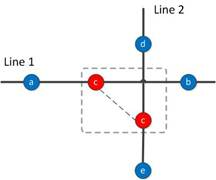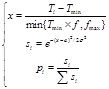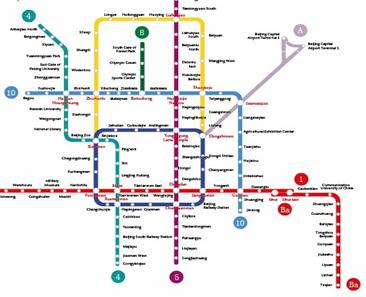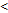Passenger route choice model and algorithm in the urban rail transit network

Passenger route choice model and algorithm in the urban rail transit network

Ke Qiao1, Peng Zhao1, Zhi-peng Qin2

1Beijing Jiaotong University, 2 The survey & design institute of railway ministry (China)

Accepted February 2013

Qiao, K., Zhao, P., & Qin Zp. (2013). Passenger route choice model and algorithm in the urban rail transit network. Journal of Industrial Engineering and Management, 6(1), 113-123. http://dx.doi.org/10.3926/jiem.595

---------------------

Abstract:

Purpose: There are several routes between some OD pairs in the urban rail transit network. In order to carry out the fare allocating, operators use some models to estimate which route the passengers choose, but there are some errors between estimation results and actual choices results. The aim of this study is analyzing the passenger route choice behavior in detail based on passenger classification and improving the models to make the results more in line with the actual situations.

Design/methodology/approach: In this paper, the passengers were divided into familiar type and strange type. Firstly passenger integrated travel impedance functions of two types were established respectively, after that a multi-route distribution model was used to get the initial route assignment results, then a ratio correction method was used to correct the results taking into account the transfer times, crowd and demand for seats. Finally, a case study for the Beijing local rail transit network is shown.

Findings: The numerical example showed that it is logical to take passenger classification and the model and algorithm is effective, the final route choice results are more comprehensive and realistic.

Originality/value: The paper offers an improved model and algorithm based on passenger classification for passenger route choice in the urban rail transit network.

Keywords: urban rail transit, route choice, travel behavior, travel impedance

---------------------

1. Introduction

With the expansion of urban rail transit network, passenger route choice has become diversified, the route choice is the basis of passenger flow assignment, and network operation and management especially fare allocating usually needs detailed passenger flow information of each route. Automatic fare collection system (AFC) can accurately get OD pairs of the entire network and the detailed information of passenger entering and leaving station. But AFC cannot accurately acquire the passenger travel route, so we need to estimate a selection probability of each route if there are several routes. There are some errors between the estimation result and the actual selection result, so we also need to improve the accuracy of the estimate. Thus, in order to solve the range of issues related to passenger flow, it is necessary to analyze the passenger route choice process in detail and compute route choice results more accurately.

In the early research of urban rail transit network assignment, some researchers learned from the road traffic flow assignment method, Wu and Liu (2004) established an equilibrium model based on equilibrium theory and resolved the model with frank-wolf algorithm. Si, Mao and Liu (2007) established passenger integrated travel impedance function and improved the equilibrium model considering the difference between road network and rail transit network. At present, with the research of fare allocating (Wei & Xie, 2009; Yang, Luo & Xu, 2009; Zhao, Zhang & Liu, 2007), the basic process of passenger flow assignment has formed in the rail transit network, firstly passenger integrated travel impedance should be computed, secondly we should compute feasible route and chose effective route, and at last we calculate the route selection probability. In the existing research, the travel impedance was expressed by generalized travel time and less considered qualitative factors such as crowd, transfer convenience and passenger familiarity on the network. Wu and Liu (2004) and Si et al (2007) put forward the calculation method of crowd, Lai (2008) used a parameter to denote passengers familiarity on the network and performed a sensitivity analysis. In term of route search algorithm, Si et al. (2007) designed a depth-first search algorithm based on graph, Liu, Sun and Bo(2009) also used the same algorithm. Xu, Luo and Gao (2009) designed delete algorithm based on depth-first algorithm and increased the travel time when judging whether the route is feasible. Improved Logit model (Liu, 2009)and probability distribution model based on normal distribution (Xu et al., 2009) are mainly used to calculate route selection probability, both models consider that the smaller the travel impedance is, the greater the probability of route will be selected is. The above-mentioned method is able to get the preliminary results of passenger flow assignment, but its accuracy has to be enhanced. In order to make the results more match the reality and improve the accuracy of models, the passenger travel impedance need to be more detailed, the qualitative factors that affect passenger route choice need to change into quantitative indicators.

Therefore, in section 2, passengers were divided the into familiar type and strange type, and two kinds of passenger integrated travel impedance function were established respectively. In section 3, the detailed method of computing route choice probability was established. Section 4 shows a numerical example for the Beijing local rail transit network.

2. Passenger integrated travel impedance

Passenger integrated travel impedance is the basic parameter of computing route choice probability and it is also the important content of network attributes abstracting. It can be described by impedance function.

2.1. Passenger classification

In the rail transit network, there are lots of factors that affect passenger route choice, the existing researches usually considered some quantitative factors such as travel time, dwelling time, transfer time and some qualitative factors such as crowd, transfer convenience. But passengers must be familiar with the network to judge these factors, they should have long-term and multiple travel experience. However, there are passengers who are shopping or touring are not familiar with the network, these passengers usually select the route by the network sketch map. So it is necessary to classify the passengers, and then calculate their travel impedance respectively.

Based on the selection basis and passenger familiarity on the network, passengers are divided into familiar type and strange type. Familiar passengers have multiple travel experience and select route by experience, such as commuters. Strange passengers rarely take the route and select route by network sketch map, such as tours and shoppers.

2.2. Integrated travel impedance of familiar passenger

Familiar passengers integrated travel impedance can be expressed by generalized travel time function including train running time, transfer time, crowd, etc. According to the existing researches, we refined transfer coefficient and considered additional entry and exit time. The travel impedance is expressed as:where,

Ca,b is the r-path travel impedance from a to b of familiar passengers;

Ti,j  is the train running time;

Ts is the train dwelling time;

Q(d) is the crowd coefficient;

Tk,walkm,n is the transfer walking time, a is the amplification coefficient;

Tk,waitm,n is the transfer waiting time, b is the amplification coefficient;

DTa is the additional entry time at original station;

DTb is the additional exit time at terminal station.

The transfer amplification coefficient was divided into transfer walking time amplification coefficient and transfer waiting time amplification coefficient. The reason is that with the equipment of transfer station updating and complete, there are lots of display screen to provide passengers abundant waiting information (current time, expected waiting time, train terminal, etc.) as well as a wealth of news, entertainment programs, this will make the waiting time passenger felt greatly reduced, so the transfer waiting time amplification coefficient decreases.

The additional entry and exit time is for special OD. When the original station (or terminal) is a transfer station, there are more entrances and exits, the time is different that the passenger from different entrance to the different platform (or from different platform to different exit). If passengers don’t choose the closest platform, it is necessary to increase some additional walking time. The additional entry time and exit time need multiplying amplification coefficient as the transfer walking time. When passengers are familiar with the route, they will consider additional entry and exit time to adjust the route selection.

2.3. Integrated travel impedance of strange passenger

The strange passengers judge and select the route by the network sketch map, so factors affecting their judgment are the straight line distance, line curve distance, number of stations and number of transfer stations in the map. The travel impedance is expressed as:where,

Tra,b is the r-path travel impedance from a to b of strange passengers;

Li,j is the straight line distance between two adjacent stations of r-path in the map;

Hi,j is the line curve distance between two adjacent stations of r-path in the map;

Nr is the number of stations except transfer stations of r-path in the map;

Mr is the number of transfer stations of r-path in the map;

g, l, m, q are the convert coefficient.

The parameter values can be obtained through the passenger survey and the proportion of familiar passengers to strange passengers needs investigating too.

3. Route choice probability computing method

Improved Logit model and probability distribution model based on normal distribution are mainly used to take passenger route choice. Luo (2009) proposed a ratio correction method of multi-route distribution model, in this paper the model was improved, firstly the initial choice probability was determined by time-based travel impedance, then the probability was corrected by transfer times, crowd and demand for seats.

3.1. Urban rail transit network representation

Urban rail transit network can be represented by the directed graph G=(V,A), in which, V={v1,v2,…vn} is the set of nodes denoting the station, A={a1,a2,…am} is the set of edges denoting the section. In passenger travel process, the transfer times has a very big impact on passenger route choice, so a transfer station should make special treatment, the approach is introducing transfer edges to make the transfer station divided to a plurality of virtual nodes belong to different lines and increasing virtual arc between these virtual nodes. A simple network example was shown in figure 1,{a, b, e, d} is not the transfer station, the red node c is a transfer station which can be divided into two virtual points belongs to Line 1 and Line 2, the dotted line shows the virtual arc, its weight is the transfer time. Through this method, we can change the actual rail network into a network graph, and then calculate the travel impedance, as the basis of route choice.Fig 1. Sketch of Transfer Node and Transfer Edge

3.2. Initial probability computational formula

According to integrated travel impedance, probability distribution model was used to take the initial route choice assignment, the model is expressed as:(3)

where,

Ti is the integrated travel impedance of i-route;

Tmin is the integrated travel impedance of the shortest route;

f is the maximum allowable increasing coefficient of travel impedance;

fmax is the maximum allowable increasing value of travel impedance;

pi is the choice probability of i-route.

3.3. The concrete computing process

The concrete computing process of route assignment is as follows:

Step 1: build urban rail transit network and determine the proportion of familiar passengers to strange passengers based on passenger surveys;

Step 2: calculate the integrated travel impedance of familiar and strange passengers according to Equation 1 and Equation 2;

Step 3: obtain OD feasible routes according to the K-short path search algorithm and determine effective route by judgment conditions. If travel impedance of a route Tk>min{(1+f), Tmin + fmax}, the route is ineffective;

Step 4: determine the choice probability of each effective route according to Equation 3;

Step 5: correct the probability through transfer times correction coefficient Xcarge. Compare transfer times between shortest route and other routes, then the initial choice probability of other routes multiply Xcarge to obtain the corrected probability and ensure the sum of probabilities is 1 at the same time;

Step 6: correct the probability through crowd correction coefficient Xcongestion. The correction method is similar to Xcarge, but this coefficient is considered only for the familiar passengers, because only it may be sufficiently familiar with the OD, it can distinguish the crowd difference between the different routes;

Step 7: correct the probability through demand for seats correction coefficient Xseats. The correction method is as above. This coefficient is for long-distance passengers, seat is an important indicator of passenger travel comfort in the long-range condition, so the passengers will first choose a route that has seats under certain threshold.

4. Computing examples

The paper proved the model having the Beijing local rail transit network for example. The network simplified schematic map is as figure 2.Fig 2. Sketch map of Beijing urban rail transit network

4.1. Parameter value

The OD from Yonganli to Jiandemen was calculated. Basis date of network derived from actual data, parameters of familiar passengers selected from Lai (2008), which are shown in table 1, different travel distance have different parameter values.

 Distance time（min） a fmax Long-distance60 1.9 15 Medial-distance 30~60 1.9 12 Short-distance30 2.3 10

Table 1. Parameter Value of Different Distance

OD from Yonganli to Jiandemen belongs to the medial OD, so the transfer walking time amplification coefficient value is 1.9. According to the section 2, the transfer waiting time amplification coefficient is less than transfer walking time amplification coefficient, the transfer waiting time amplification coefficient is 1.5. Therefore the parameter values of familiar passengers are shown in table 2.

 Parameter a b fmax value 1.9 1.5 12

Table 2. Parameter Value of Familiar Passengers

According to the Beijing Urban Rail Transit AFC data, the proportion of passengers holding a long-term card to passengers holding the temporary card is approximately 2:1, assuming that passengers holding long –term card are familiar passengers and passengers holding the temporary card are strange passengers, this paper set that strange passengers ratio is 33% and familiar passengers ratio is 67%. According to the actual survey results, parameter values of strange passengers are shown in Table 3.

 Parameter m g l q value 0.4 2.5 2 2.9

Table 3. Parameter Value of Strange Passengers

4.2. Computing result

Firstly, the integrated travel impedance was computed and the route effectiveness was judged, as shown in table 4.

 Route Travel impedance Effectiveness Number Route details Passenger type Value 1 Yonganli-Dongdan-Huixinxijienan kou- Jiandemen familiar 42.8 effective strange 34.7 effective 2 Yonganli-Guomao-Jiandemen familiar 37.2 effective strange 36.7 effective 3 Yonganli-Jianguomen-Yonghegong-Huixinxijienankou-Jiandemen familiar 51.4 ineffective strange 36.9 effective 4 Yonganli-Jianguomen-Dongzhimen-Shaoyaoju-Jiandemen familiar 74.3 ineffective strange 40.8 effective

Table 4. OD Travel Routes Choice

According to the effective route impedance of different passengers, the initial route assignment result was calculated by probability distribution models. This case mainly considered the correction of transfer times. The result is shown in table 5. Combined with the proportion of different types of passengers, the final route assignment result is shown in Table 6.

 Passenger type Route Computing result Transfer times Correction coefficient Correction result Familiar 1 14.88% 2 0.7 10.42% 2 85.12% 1 - 89.58% Strange 1 37.16% 2 - 31.03% 2 29.75% 1 1/0.7 42.5% 3 28.39% 3 0.8 22.71% 4 4.70% 3 0.8 3.76%

Table 5. Initial Calculation Result and Correction Result

 Route Passenger type Passenger ratio Computing result Final Result 1 Familiar 67% 10.42% 17.22% Strange 33% 31.03% 2 Familiar 67% 89.58% 71.63% Strange 33% 42.5% 3 Familiar 67% 0 7.49% Strange 33% 22.71% 4 Familiar 67% 0 1.24% Strange 33% 3.76%

Table 6. Final Calculation Result

The above is the calculation process of passenger route choice from Yonganli to Jiandemen. It can be seen that, route 3 and route 4 are ineffective for familiar passengers, but are effective for strange passengers who judge the route by map. Indeed there will be a certain percentage of passengers choose route 3 and route 4, this showed taking passengers classification can get more comprehensive results, it is logical to take passenger classification and the final results are more realistic.

In addition, the initial ratio of route 2 is less than the route 1 for strange passengers, it seems that the ratio is not realistic, but after the transfer times correction, the ratio of route 2 increased, this is closer to actual choices, so coefficient correction is an effective method.

5. Conclusions

According to passenger familiarity on the network, passengers were divided into two types: familiar type and strange type. The different integrated travel impedance function for two types of passengers was set up. For the familiar passengers, the travel impedance increased additional entry and exit time; the transfer amplification coefficient was refined to make the function more realistic. For the strange passengers, the travel impedance was mainly based on the rail transit network map. The ratio correction method of multi-route distribution model was used to take route choice. The results can be corrected by coefficient of crowd, coefficient of transfer times and coefficient of demand for seats. The case of Beijing local rail transit network showed that the passenger route choice behavior can be depicted accurately through classifying the passengers.

Acknowledgments

This work was financially supported by The National Key Technology R&D Program (2009BAG12A10) from Ministry of Science and Technology of P.R.China.

References

Lai, S.K. (2008). Research on income distribution model of urban rail transit. Unpublished master’s thesis, Beijing Jiaotong University, Beijing.

Liu, J.F. (2010). Passenger flow distribution model of urban rail transit network based on data obtained IC card usage. Logistics Technology, 8, 64-67.

Liu, J.F., Sun, F.L., & Bo, Y. (2009). Passenger flow route assignment model and algorithm for urban rail transit network. Journal of Transportation Systems Engineering and Information Technology, 5, 131-133.

Luo, Q. (2009). Theory and simulation analysis of passenger flow distribution based on network operation for urban mass transit. Unpublished doctoral dissertation, Tongji University, Shanghai.

Si, B.F., Gao, L., & Mao, B.H. (2009). Toll allocation model for urban railway traffic system under condition of seamless exchange. Journal of Management Sciences in China, 12(5), 36-43.

Si, B.F., Mao, B.H., & Liu, Z.L. (2007). Passenger flow assignment model and algorithm for urban railway traffic network under the condition of seamless transfer. Journal of the China Railway Society, 29(6), 12-18.

Wei, Q., & Xie, Z.Y. (2009). Fare clearing algorithm based on probabilistic model for urban Rail transit. Urban Mass Transit, 9, 43-47.

Wu, X.Y., & Liu, C.Q. (2004). Traffic equilibrium assignment model specially for urban railway network. Journal of Tongji University (Natural Science), 32(9), 1158-1162.

Xu, R.H., Luo, Q., & Gao, P. (2009). Passenger flow distribution model and algorithm for urban rail transit network based on multi-route choice. Journal of the China Railway Society, 31(2), 110-114.

Yang, J., Luo, Q., & Xu, R.H. (2009). A clearing method of AFC on urban mass transit. Urban Mass Transit, 5, 22-25.

Zhao, F., Zhang, X.C., & Liu, Z.L. (2007).  Modelling income distribution of the auto fare collection system. Journal of Transportation Systems Engineering and Information Technology, 7(6), 85-90.